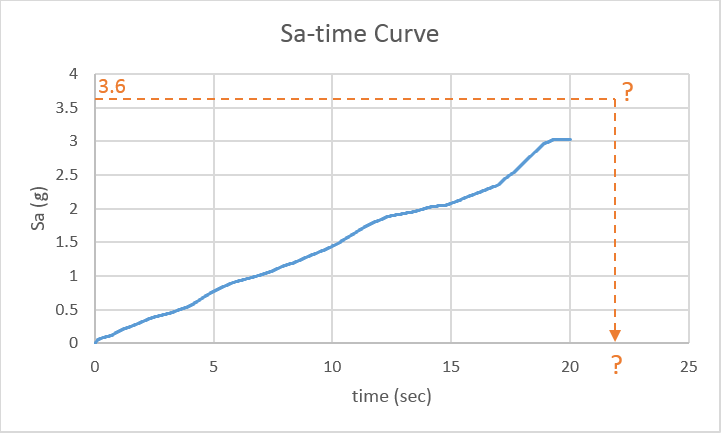# Estimating the Average and Standard Deviation with Missing Data

#### rosicky

##### New Member
I need a way to shows me how the parameters of PDF, log-normal in this case, can be estimated based on a set with missing data points at the tail end of a sample.

For example, Consider we had 20 numbers with specified μ and σ, and then missed two largest number of them. How do we can estimate the mean (μ) and standard deviation (σ) of these 20 numbers with only 18 available numbers, if we know that all of them obey a log-normal distribution?! (this is obvious that these 2 missing numbers are in the tail end of log-normal distribution)

*Please don't present complex solutionsI want to code a program in Matlab to do this for me.

#### Dason

So it wasn't just two random values from the sample that got dropped? It was the two largest values? That does make the solution more complex.

#### noetsi

##### No cake for spunky
If you are doing some type of multivariate analysis the answer would be multiple imputations. I am curious how you would know that two values are missing (why not three or one or four)?

You can add two values from a known distribution through simulation. You can specify that the answers being filled in are so many standard deviations from the mean. But there is no way to be sure that this is the unknown mean and standard deviation, it is only a plausible mean and standard deviation. The only way you could know the correct results is if you know how many values are missing and their level.

#### rosicky

##### New Member
yes, largest values! my thesis has stopped just for this problem#### noetsi

##### No cake for spunky
How do you know that there are exactly two values missing and they are in the tail?

#### Dason

Do you know about maximum likelihood estimation?

#### rosicky

##### New Member
The main problem is that I have some values from a parameter which is named "Sa" (see below fig). I want to get the equivalent "time" for each "Sa" from this curve. But some of the values ("Sa") are out of curve domain! So I know how many values are missing.Then, I suppose that all of "time" values with missing data obey a log-normal distribution!
(I think, regression methods is not appropriate for my problem)

#### hlsmith

##### Less is more. Stay pure. Stay poor.
Do you have any other data available for these observations that can help deduce there value, beyond that they are the 2 largest?